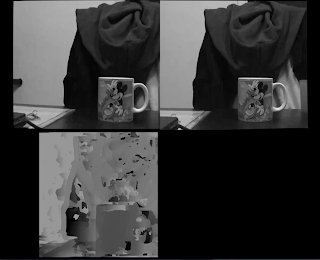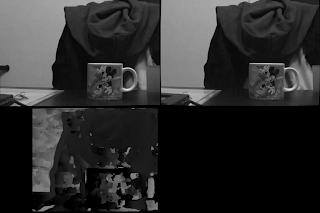## Wednesday, January 1, 2014

### Better Stereo (?) using Rectified Left and Right images and stereo Q matrix with and without zero disparityLeft and right images with zero disparity calibration and the resulting disparity map. With higher possible disparity values (this is a paremeter for the alogithm), the left of the map is black, but gives better results in general for the found disparity.
So the stereo camera is important to provide 3D information of the world that is currently visible. The difference in position of an object in the left and right images defines the objects position. With this information we can start reconstructing the world around the camera. So there are two points:
1.) Determining the relative position of objects in the left and images in terms of how many pixels the object's position is different from left to right. This is done using something like stereo block matching.
2.) Translate this pixel difference in to real world values like centimeters and meters using the camera parameters.

Before 1 and 2 can be done, the left and right images need to be rectified to fit into the normalized camera reference point, so the algorithms can correctly run. See references on epipolar lines or rectified stereo images.

The one issue I ran into was how was the Q matrix in 2 above defined. ScaViSLAM uses a Q matrix with "zero disparity" this means that the Q matrix looks like this (see StereoCamera::Q()):
1    0    0    Cx
0    1    0    Cy
0    0    0     f
0    0    1/b  0

where (Cx, Cy) is the camera center point, f is the camera focal distance and b is the baseline distance (distance between the left and right camera center points). To obtain this value using openCV function cvStereoRectify, you must use the CV_CALIB_ZERO_DISPARITY flag. If you don't use this then your matrix looks like:

1    0    0    Cx
0    1    0    Cy
0    0    0     f
0    0    1/b  b'/b

Where b' is the shift between the 2 cameras. Using a non zero disparity should help to conserve pixel area after rectification. But essentially both should work, its just a question of what the different algorithms are expecting. ScaViSLAM uses a hierarchy of images, so recalculates the camera parameters for each scale, which it then needs the zero disparity matrix. You can do the same calculations for non-zero disparity, but it was easiest to get started using the CV_CALIB_ZERO_DISPARITY flag.Left and right images with non-zero disparity calibration and the resulting disparity map. More area of the captured images is preserved. The disparity map looks quite difference due to the different Q matrix that will reproject the points

I cam across this blog: http://robotpingpong.blogspot.de/2013/03/small-progress-in-triangulation.html. Here the results for the rectification are much more warped. I guess because his 2 PS3 cameras are not so closely arranged. The raspberry pi camera gives good results here because you can mount then closely (6.5cm apart). His cameras I think were 50cm apart.

### Working with raspivid for low latency and other issues with compression

So after I got ScaViSLAM working, I had an issue with getting my raspi stereo camera working at 640x480 as expected (I wanted to use 640x480 because the perf at 1920x1080 was too slow, but the perf would probably get better in a release build). The issue was that I was getting bursts of 17 frames in the stream. Instead of the h264 stream from the pi delivering one frame as often as possible, it was sending 17 frames in one packet. This meant that there was a latency of 33ms*17=500ms. This is not so usable. I eventually analyzed the H264 stream by detecting the NAL markers, which showed that the H264 decoder was decoding 17 frames for a I frame packet (buffer starting with 0x00000125). An I frame is a single frame that is only compressed against itself, there is no dependency to previous frames. A good reference for the H264 basics is
http://keyj.emphy.de/files/projects/SA_paper.pdf. I don't know if another decoder could handle this better or if this is normal, but then the solution was to increase the frequency of the I frames. using "raspivid -g 1" the I frames are then sent every frame. Then the problem is solved because then I receive as new a frame as fast possible and my video queue is always at most 1 deep (unless whatever algorithm that is running on the server is too slow to consume the stream).

I added code in
https://github.com/arcanon/raspbot/blob/master/h264decoder.h
to detect the NAL markers and also check how full the frame queue was.

```
if ((data[i] == 0) &&

(data[i+1] == 0) &&

(data[i+2] == 0) &&

(data[i+3] == 1))

{

NALCount++;

nalFound = true;

....

}
and

if ((m_reader->framesInQueue()- start) > 1)

{

falseCount+= (m_reader->framesInQueue()- start);

printf("%s, error frames more %d size %d fa %d, nc %d fc %d\n", this->m_vid_server.c_str(), (m_reader->framesInQueue()- start), prevSize, framesAdded, NALCount, falseCount);    }

```